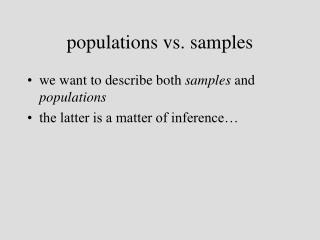Download Presentationpopulations vs. samples

# populations vs. samples - PowerPoint PPT PresentationDownload Presentation## populations vs. samples

- - - - - - - - - - - - - - - - - - - - - - - - - - - E N D - - - - - - - - - - - - - - - - - - - - - - - - - - -
##### Presentation Transcript

1. populations vs. samples • we want to describe both samples and populations • the latter is a matter of inference…

2. “outliers” • minority cases, so different from the majority that they merit separate consideration • are they errors? • are they indicative of a different pattern? • think about possible outliers with care, but beware of mechanical treatments… • significance of outliers depends on your research interests

3. summaries of distributions • graphic vs. numeric • graphic may be better for visualization • numeric are better for statistical/inferential purposes • resistance to outliers is usually an advantage in either case

4. general characteristics [“peakedness”] • kurtosis ‘leptokurtic’ ’platykurtic’

5. right(positive) skew left(negative) skew • skew (skewness)

6. central tendency • measures of central tendency • provide a sense of the value expressed by multiple cases, over all… • mean • median • mode

7. mean • center of gravity • evenly partitions the sum of all measurement among all cases; average of all measures

8. mean – pro and con • crucial for inferential statistics • mean is not very resistant to outliers • a “trimmed mean” may be better for descriptive purposes

9. mean R: mean(x)

10. trimmed mean R: mean(x, trim=.1)

11. median • 50th percentile… • less useful for inferential purposes • more resistant to effects of outliers…

12. median

13. mode • the most numerous category • for ratio data, often implies that data have been grouped in some way • can be more or less created by the grouping procedure • for theoretical distributions—simply the location of the peak on the frequency distribution

14. 1.0 1.5 2.0 2.5 modal class = ‘hamlets’ isolated scatters hamlets villages regional centers regional centers

15. dispersion • measures of dispersion • summarize degree of clustering of cases, esp. with respect to central tendency… • range • variance • standard deviation

16. would be better to use midspread… range R: range(x)

17. R: var(x) variance • analogous to average deviation of cases from mean • in fact, based on sum of squared deviations from the mean—“sum-of-squares”

18. variance • computational form:

19. note: units of variance are squared… • this makes variance hard to interpret • ex.: projectile point sample: mean = 22.6 mm variance = 38 mm2 • what does this mean???

20. standard deviation • square root of variance:

21. standard deviation • units are in same units as base measurements • ex.: projectile point sample: mean = 22.6 mm standard deviation = 6.2 mm • mean +/- sd (16.4—28.8 mm) • should give at least some intuitive sense of where most of the cases lie, barring major effects of outliers